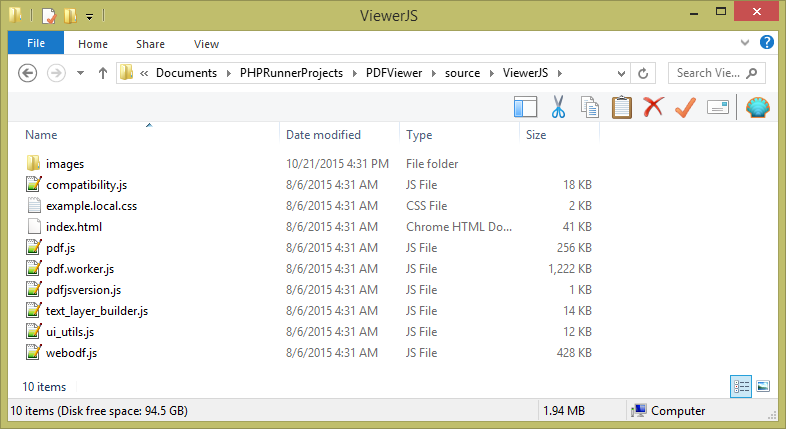In recent Using Google Docs Viewer to preview documents online article we reviewed the use of Google Docs Viewer for document preview purposes. While this is a viable approach two main concerns arise:

• dependency on third party service
• security issues

For those who is looking for another solution there is a brilliant Javascript library named ViewerJS that supports PDF files as well as Open Office formats (.odp, .odt, .ods). This library can display documents right in the web browser, no need to install any additional software. Documents can be displayed either in full page mode or embedded into other pages. See some examples.

In this article we’ll show how you can use ViewerJS library in your PHPRunner projects. Before you do that feel free to check the live demo.

Here are integration steps:2. Setup one of fields as document upload. You can use either basic upload or multiple-files upload. Set ‘View as’ type of this field to ‘Custom’ and use the following code.

PHP code:

```\$mftable = "carscars";
\$mfield = "descr";
\$ext=strtoupper(substr(\$value,strlen(\$value)-4));
if(\$ext == ".PDF")
\$value="<a target='_blank' href='ViewerJS/#../mfhandler.php?file=".\$value."&table=".\$mftable."&field=".\$mfield."&pageType=list&key1=".\$data["id"]."'>".\$value."</a>";
else
\$value="<a target='_blank' href='mfhandler.php?file=".\$value."&table=".\$mftable."&field=".\$mfield."&pageType=list&key1=".\$data["id"]."' dir='LTR'>".\$value."</a>";
```

C# code:

```dynamic ext = null, mfield = null, mftable = null, value = null;
mftable = new XVar("carscars");
mfield = new XVar("descr");
ext = XVar.Clone(CommonFunctions.strtoupper((XVar)(CommonFunctions.substr((XVar)(value), (XVar)(MVCFunctions.strlen(value) - 4)))));
if(ext == ".PDF")
{
value = XVar.Clone(MVCFunctions.Concat("", value, ""));
}
else
{
value = XVar.Clone(MVCFunctions.Concat("", value, ""));
}
return null;
```

In this code \$mftable is the name of the table, \$mfield is the name of the field that stores documents.

PHP code:

```\$arrfile = my_json_decode(\$value);
\$value="";
\$mftable = "carscars";
\$mfield = "descr";
for(\$i=0;\$i < count(\$arrfile);\$i++){
if(\$value)
\$value.=", ";
if(\$arrfile[\$i]["type"] == "application/pdf")
\$value.="<a target='_blank' href='ViewerJS/#../mfhandler.php?file=".\$arrfile[\$i]["usrName"]."&table=".\$mftable."&field=".\$mfield."&pagetype=list&key1=".\$data["id"]."'>".\$arrfile[\$i]["usrName"]."</a>";
else
\$value.="<a href='mfhandler.php?file=".\$arrfile[\$i]["usrName"]."&table=".\$mftable."&field=".\$mfield."&pagetype=list&key1=".\$data["id"]."' dir='LTR'>".\$arrfile[\$i]["usrName"]."</a>";
}
```

C# code:

```dynamic arrfile = XVar.Array(), i = null, mfield = null, mftable = null, value = null;
arrfile = XVar.Clone(CommonFunctions.my_json_decode((XVar)(value)));
value = new XVar("");
mftable = new XVar("carscars");
mfield = new XVar("descr");
i = new XVar(0);
for(;i < MVCFunctions.count(arrfile); i++)
{
if(XVar.Pack(value))
{
value = MVCFunctions.Concat(value, ", ");
}
if(arrfile[i]["type"] == "application/pdf")
{
value = MVCFunctions.Concat(value, "", arrfile[i]["usrName"], "");
}
else
{
value = MVCFunctions.Concat(value, "", arrfile[i]["usrName"], "");
}
}
return null;
```

Some additional bits of info. This sample code will display PDF files only, other files simply be downloaded to the end user device. This code is designed to open document preview in new window. If you like to open it in the same window remove target=_blank from the code.

## 1 thought on “Adding PDF preview support to your web application”

1.Matthias says:

Hi,

Question, what is the trick behind if my pdf’s not located at the web root folder?

Thank you.

Matthias